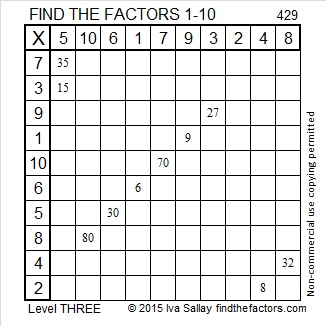# Level 3 and Divisibility Tricks Applied to 429

Today my blog has had 42 referral views from twitter, mostly thanks to other people’s tweets. Thank you for the tweets and retweets. Also, welcome everyone who visits my blog because of them or for any other reason!Print the puzzles or type the factors on this excel file:10 Factors 2015-03-16

Let’s try some divisibility tricks on the number 429:

4 + 2 is 6, a multiple of three, so 429 is a divisible by three. Why didn’t I include 9 in the sum? Because I already know that 9 is a multiple of three, so it is redundant to include it!

Now try another divisibility trick on 429: I’ve put the odd numbered digits in bold and the even numbered digits in regular type. If we sum the odd numbered digits and subtract the even numbered digit, we get 4 + 9 – 2 = 11, a multiple of 11. That means that 429 is also divisible by 11!

• 429 is a composite number.
• Prime factorization: 429 = 3 x 11 x 13
• The exponents in the prime factorization are 1, 1, and 1. Adding one to each and multiplying we get (1 + 1)(1 + 1)(1 + 1) = 2 x 2 x 2 = 8. Therefore 429 has exactly 8 factors.
• Factors of 429: 1, 3, 11, 13, 33, 39, 143, 429
• Factor pairs: 429 = 1 x 429, 3 x 143, 11 x 39, or 13 x 33
• 429 has no square factors that allow its square root to be simplified. √429 ≈ 20.7123A Logical Approach to solve a FIND THE FACTORS puzzle: Find the column or row with two clues and find their common factor. Write the corresponding factors in the factor column (1st column) and factor row (top row).  Because this is a level three puzzle, you have now written a factor at the top of the factor column. Continue to work from the top of the factor column to the bottom, finding factors and filling in the factor column and the factor row one cell at a time as you go.